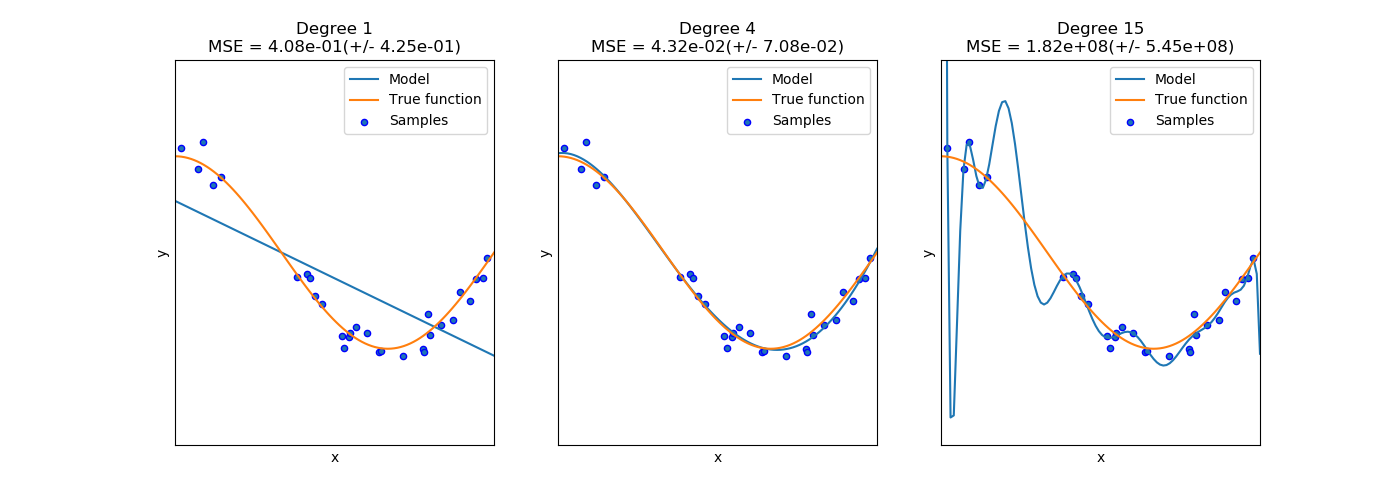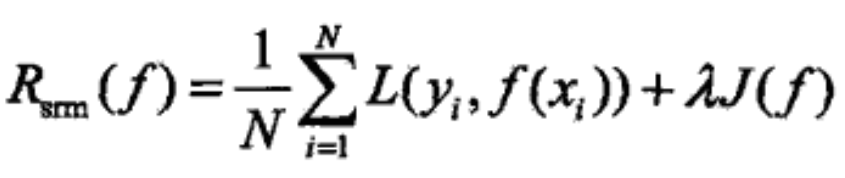# Empirical & Structural Risk Minimization

• 0–1 Loss: L(Y, f(X)) = 1, if Y ≠ f(X) or 0 if Y = f(X),
• Quadratic Loss: L(Y, f(X)) = (Y−f(X))²
• Log-Likelihood Loss: L(Y,P(Y|X)) = −logP(Y|X)Empirical Risk. Credit: https://en.wikipedia.org/wiki/Empirical_risk_minimizationFitting the data points by polynomial functions with different degree. The right-most plot shows the classifier fit the data points perfectly while the curve is obviously deviated from the true function which we want to fit. Credit: http://scikit-learn.org/stable/auto_examples/model_selection/plot_underfitting_overfitting.html.Structural Risk. Credit: 李航《統計學習方法》

--

--

## More from Meng-Jiun Chiou

Computer Vision Applied Scientist @ Amazon. https://coldmanck.github.io

Love podcasts or audiobooks? Learn on the go with our new app.